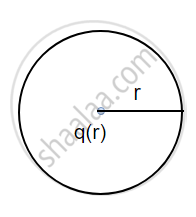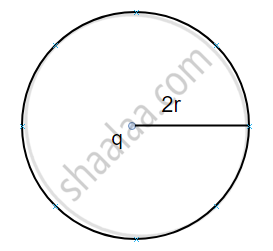# State Gauss'S Law in Electrostatics. Show, with the Help of a Suitable Example Along with the Figure, that the Outward Flux Due to a Point Charge 'Q'. in Vacuum Within a Closed Surface - Physics

State Gauss's law in electrostatics. Show, with the help of a suitable example along with the figure, that the outward flux due to a point charge 'q'. in vacuum within a closed surface, is independent of its size or shape and is given by q/ε_0

#### Solution

Statement: The electric flux linked with a closed surface is equal to (1)/ε_0 times the net charge enclosed by a closed surface.

Mathematical expression :

Ø_"E" = oint  vec"E".dvec"s" = (1)/(ε_0) (q_"net")

Consider two spherical surfaces of radius r and 2r respectively and a charge 1 is enclosed in it. According to gauss theorem, the total electric flux linked with a closed surface depends on the charge enclosed in it so

(a)(b)Ø_E = q/ε_0 "and for fig"("b")

Ø_E = q/ε_0.

Concept: Gauss’s Law
Is there an error in this question or solution?
2014-2015 (March) Ajmer Set 2

Share